# From Sample & Hold To Sample-rate Converters (2)

Synth Secrets By Gordon Reid
Published September 2000

Sample & Hold modules, as explained last month, convert a continuously varying signal into a stepped series of fixed pitches. And this, as we shall see, is the basis of what we know as 'digital audio'...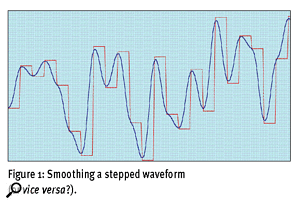Figure 1: Smoothing a stepped waveform (or vice versa?).Do you remember Figure 1? It featured in last month's Synth Secrets, and when I presented it to you, I asked the following question: Is the red line the input from an S&H module, with the blue line the smoothed output? Alternatively, is the blue line an audio signal input, with the red line the S&H output? This month we're going to find out the answer.

## Generating Step Sequences

In Synth Secrets Part 16 (SOS August 2000) we talked a lot about Sample and Hold modules and step sequencers, two devices that generate 'stepped' CV signals at their outputs. So let's start here, and consider the 13-step sequencer shown in Figure 2. OK, I admit that I've never heard of a 13-step sequencer, but that's not relevant — most 16-step devices allow you to truncate the sequence at any step you wish. Anyway, let's set up our sequencer as shown in Figure 3...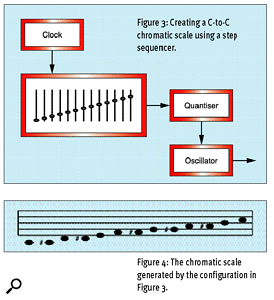Figure 3: [top] Creating a C-C chromatic scale using a step sequencer. Figure 4: [bottom] The chromatic scale generated by the configuration in Figure 3.I have set the faders on the sequencer so that (hypothetically) it outputs 1V at step one, 1.083V at step two, 1.167V at step three, and so on up to precisely 2V at step 13. The eagle-eyed among you will notice that this quantised output represents a chromatic scale from C1 to C2 on a 1V/octave synthesizer. To ensure that the output conforms precisely to these voltages, I have inserted a quantiser between the sequencer and the oscillator it controls. We can then represent the output as shown in Figure 4.

Now, let's hypothesise that the clock runs at one pulse per second. Clearly, the oscillator will step between notes once per second, completing the scale once every 13 seconds before resetting to the beginning and starting again. But what happens if we accelerate the clock? At 130 pulses per second, the scale repeats 10 times per second, creating a complex tone at the output.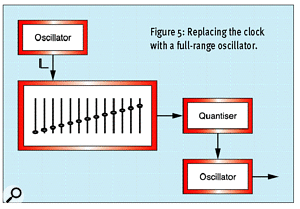Figure 5: Replacing the clock with a full-range oscillator.If you try to accelerate a conventional synthesizer clock much further, you'll soon reach its limit. This is because clocks are low-frequency oscillators, most of which have a maximum frequency of just a few hundred Hertz. So let's replace the dedicated clock with an audio-frequency oscillator. If we set this to a tight pulse waveform, there's no reason why it shouldn't act like a clock, but with a far wider range of frequencies. See Figure 5.

If we now increase the trigger speed (ie. the oscillator frequency) and continue to direct the sequencer's output to another oscillator, we might soon obtain a scream of protest at the output. At a trigger frequency of 13000Hz, the scale repeats 1000 times per second. If you have a high-quality synthesizer, you might hear this as another complex tone. Instruments with lesser electronics will honour you with silence, perhaps because the clock oscillator can't produce a tight enough pulse at such a high frequency, or because the sequencer can't handle such a rapid trigger, or even because the quantiser can't respond at these speeds.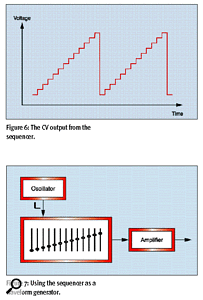Figure 6: [top] The CV output from the sequencer. Figure 7: [bottom] Using the sequencer as a waveform generator.But let's now look at the quantiser's output in a different way. Figure 6 shows the idealised CV waveform that it produces. It looks a bit familiar, doesn't it? Clearly, it's a 'staircased' version of the common sawtooth wave. So what happens if we take this and, instead of using it to control another module, we treat it as a raw audio signal? The configuration is shown in Figure 7. (For simplicity, I've discarded the quantiser and assumed that the sequencer produces precisely the voltages that we request.)

The result, as you might imagine, is a sawtooth(-ish) waveform, with a frequency of 1/13th that of the trigger oscillator. Indeed, it's even more like a sawtooth than you might imagine because no sequencer can jump instantaneously between voltages, and no amplifier has infinite bandwidth. These limitations smooth the 'steps' in the waveform (see Figure 8). You might say that the output is not really a sawtooth wave, and you would be right — there is a higher proportion of high harmonics than you might otherwise expect. However, it's at least as accurate as that produced by many dedicated oscillators.

Let's now return to the idealised situation, and use the sequencer to generate some more waveforms (see Figures 9, 10 and 11). Analysing Figure 9 is easy: it's a 12-step sequence with six steps high and six steps low. Clearly, I'm using the sequencer to generate a square wave. Figure 10 is similarly straightforward: it's a pulse wave with a duty cycle of 1:3. But what about Figure 11? This isn't a waveform that you'll see on a conventional analogue oscillator. It is, however, one that you'll see generated by (hush please... sacrilege alert!) a digital oscillator.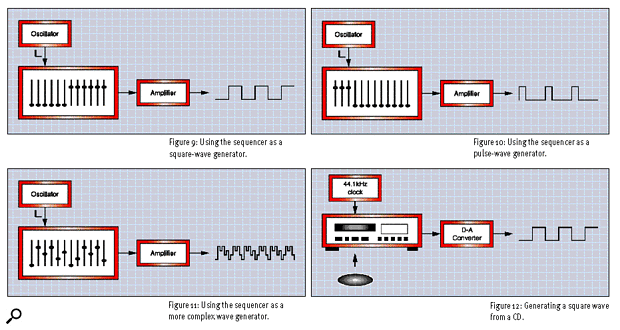Figures 9 [top-left] Using the sequencer as a square-wave generator. Figure 10: [top-right] Using the sequencer as a pulse-wave generator. Figure 11: [bottom-left] Using the sequencer as a more complex wave generator. Figure 12: [bottom-right] Generating a square wave from a CD.

You may not realise it, but we've come to a turning point in our understanding of analogue versus digital synthesis. I'm sure that you had no difficulty coming to terms with the fact that we can perform FM synthesis on an analogue synthesizer (Synth Secrets, SOS April and May 2000). Similarly, you probably had no problem accepting that we can perform simple additive synthesis on even a moderately endowed modular analogue (Synth Secrets, SOS June 2000). But now I'm going to ask you to accept that we can use our understanding of S&H, slewing, and step sequencers to describe the very fundamentals of digital audio.

## Analogue Or Digital?

Figure 9 (above) depicts an ideal square wave generated by a 'perfect' analogue step sequencer. Figure 12 also shows an ideal square wave, but this time it's generated by a CD player reading the digital data held on a CD. To explain why this is relevant, let's remind ourselves about what's happening in the CD player. A bunch of samples are held as binary numbers on the CD itself and, on playback, one sample is read and presented to a digital-to-analogue converter (DAC) each 1/44100th of a second.

If we keep everything simple, and ignore detailed discussions of the coding used in CD technology, we could say that the 'low' part of the square wave is stored as binary 0000000000000000, a 16-bit sample of minimum amplitude. Likewise, we could say that 1111111111111111, a 16-bit sample of maximum amplitude, represents the 'high' part of the square wave. Let's now postulate that we have six 'low' samples followed by six 'high' samples, and that this pattern repeats itself for the duration of the CD. The result is a constant square wave with a frequency of (44100/12) Hz — which is 3675Hz.

Let's now return to the ideal analogue sequencer in Figure 9, and (using a suitably rapid clock) trigger it 44,100 times per second. The result is... a constant square wave with a frequency of (44100/12) Hz — which is 3675Hz. Clearly, within the limits of the electronics employed, the results are identical.

Likewise, we can use our CD player to reproduce the waveforms shown in Figures 10 and 11, simply by storing and replaying appropriate samples on a CD. This implies that we can also store and replay synth solos, drum loops, the Vienna Philharmonic playing Beethoven's 6th Symphony... or anything else, provided that we can represent the amplitude of the original signal using a succession of binary numbers. So, where do these 'digital' samples come from?

## Analogue To Digital

Let's return to Synth Secrets 16, and our discussion of Sample and Hold circuits. Figure 13 shows what happens when we sample a sine wave approximately six times every cycle. The output waveform looks very 'blocky', but what is important at this stage is that we have taken a continuous waveform and reduced it to a small number of discrete measurements. We can then pass these measurements to another stage within an analogue-to-digital converter (ADC) that accepts each voltage sample in turn, converts it to a binary number, and sends the number to an appropriate storage medium.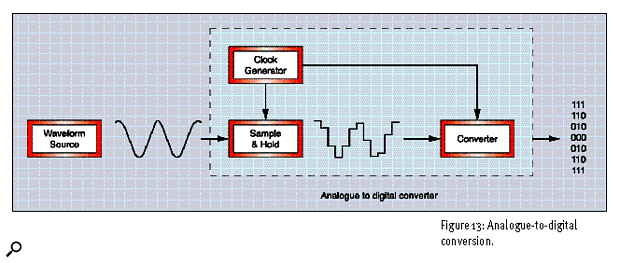Figure 13: Analogue-to-digital conversion.

Of course, nothing is ever that simple, so we must consider the limitations of the digitising process. Ignoring the inadequacies of real-world electronics, there are two major sources of limitation — one related to amplitude, the other to timing.

Let's look at the first of these. It's simply this: we cannot sample the voltage of the incoming signal with infinite resolution — there is a limit to the number of ways in which we can chop up the amplitude.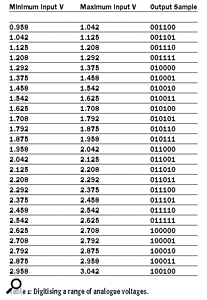Table 1: Digitising a range of analogue voltages.If you think that, at this point, we're a million miles from discussions of analogue synthesizers, S&Hs and sequencers, you're wrong. Look at Table 1, parts of which I've copied from last month's Synth Secrets. Previously, I used these values to illustrate voltage quantisation, demonstrating how a quantiser will accept any voltage lying between a given maximum and minimum, and output the central value of that band. Instead of outputting a quantised voltage, the ADC takes any voltage lying between a given maximum and minimum, and outputs a unique binary number that represents the central value of that band (see Figure 14). Sure, last month's context was different, but the two processes are conceptually very similar. The major difference is, perhaps, that last month we chose to quantise the levels, whereas this month we have no choice, because digital numbers of finite word-length can only represent a finite number of levels.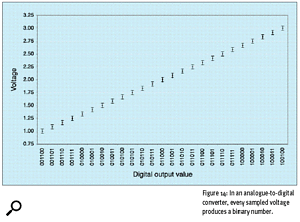Figure 14: In an analogue-to-digital converter, every sampled voltage produces a binary number.The second limitation in analogue-to-digital conversion concerns the sampling frequency of the system: just as we can not digitise the amplitude with infinite precision, we can not conduct the measurements with infinite speed.

We now arrive at the most important theorem in digital audio. This is the Shannon-Nyquist Sampling Theorem, named in honour of the two gentlemen who demonstrated and proved its veracity. (The Theorem is not specific to digital audio, but applies to any sampled signal.) You'll be pleased to know that proving the Theorem is so far from trivial that I won't even attempt to show it here. However, I can easily state its most important tenet, as follows:

If a signal has a bandwidth of less than F (the Nyquist frequency), then 2F samples per second are sufficient to represent that signal fully and unambiguously.

There are a couple of hidden conditions in this statement. (The first is that a limited bandwidth implies a signal of infinite duration, while the second is that you must sample the analogue signal at evenly spaced intervals.) But we are not going to worry about these. Instead, we're going to look at its most important consequence:

An analogue signal can be reconstructed, without error, from samples taken at equal time intervals, provided that the sampling rate is greater than twice the highest-frequency component in the original signal.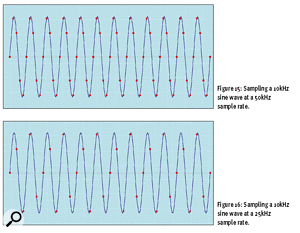Figure 15: [top] Sampling a 10kHz sine wave at 50kHz sample rate. Figure 16: [bottom] Sampling a 10kHz sine wave at a 25kHz sample rate.It's possible to demonstrate this pictorially. Look at Figure 15. This shows what happens when you sample a sine wave at exactly five times its frequency. If, for the sake of argument, the waveform has a frequency of 10kHz, this represents a sampling rate of 50kHz, and it should be obvious that this is more than sufficient to describe the sine wave accurately. Figure 16 depicts the same situation, except that I have reduced the sampling rate to 25kHz. As you can see, the samples are more widely spaced, but they remain sufficient to define the waveform unambiguously.

Figure 17 demonstrates a completely different state of affairs. The samples still lie on the input signal, but they now depict a waveform (the orange line) that completes one cycle for every three cycles of the original. This means that there is, in principle, a different frequency — 3.33kHz — in the sampled data. How has this happened?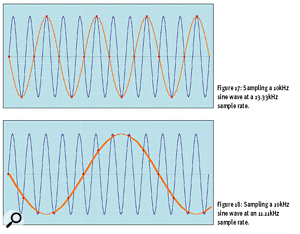Figure 17: [top] Sampling a 10kHz sine wave at a 13.33kHz sample rate. Figure 18: [bottom] Sampling a 10kHz sine wave at an 11.11kHz sample rate.The answer lies in an effect called 'aliasing'. I'm sure that you have encountered this name before, especially if you own an FM synthesizer, but maybe you've never known exactly what it is. So here's the explanation. The sample rate in Figure 17 is 13.33kHz, and the Nyquist Theorem tells us that the maximum frequency that this can accurately represent is 6.67kHz. Since this sample rate cannot handle our 10kHz input, a strange effect occurs: any frequencies above the Nyquist frequency 'fold over' and appear an equal distance below the Nyquist frequency. The high frequencies reappear 'under an alias'. In this example:

• The Nyquist frequency = 6.67kHz.
• The input signal = 10kHz.
• The 'aliased' frequency is (10kHz minus 6.67kHz, subtracted from 6.67kHz) = 3.33kHz.

Figure 18 (above) makes this effect even more obvious. This shows our 10kHz source sampled at just 11.11kHz, and you can see clearly that the samples, which still lie on the source waveform, now describe a wave of 1.11kHz.

Unfortunately, you can't remove aliasing once it has been introduced, so we have to ensure that it never occurs. We do this by making sure that there are no frequencies above 'F' in the analogue signal presented to the ADC. How? By sticking a low-pass filter in the signal path. However, this filter introduces more problems — in particular, the detrimental phase shifts described in Synth Secrets Part 4 (SOS August 1999). When you analyse it, all this digital stuff is very analogue!

## Digital To Analogue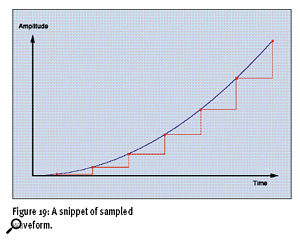Figure 19: A snippet of sampled waveform.Once we've digitised our audio, we still need to convert it back to analogue in order to hear it. So, how do we get back to the original using our samples as a starting point? Let's forget all about audio — analogue or digital — for a moment, and return to the schoolroom. It's 2:30pm and you're stuck in double maths. Yurg! Up at the front of the class, your teacher has drawn a curve, together with the common graphical representation for a set of seven samples taken along its length (see Figure 19).

Given these samples, I'm going to tell you that I know the exact amplitude of the curve at every moment along the 'time' axis. How? Well, look at Table 2, which shows the values of the samples in Figure 19.

If you think back to your school maths, you'll recognise what I've done. The curve is a parabola, with the amplitude of any sample equal to the square of the Time. We write this as Equation 1.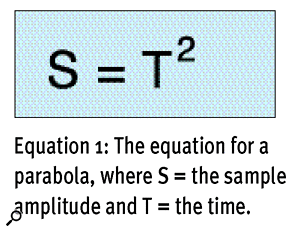Equation 1: The equation for a parabola, where S = the sample amplitude and T = the time.

Equation 1: The equation for a parabola, where S = the sample amplitude and T = the time.

It looks simple, and it is. Nevertheless, it hides a marvellous secret. Whereas the samples in Figure 19 were 'taken' at integer times (2, 5, 8, and so on) the equation allows us to calculate the amplitude at any time we like within the span of the given samples. This could be another integer time, but it could also be at T = 3.6347563356, or whenever else we choose.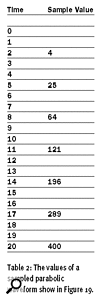Table 2: The values of a sampled parabolic waveform shown in Figure 19.At this point, the cynical among you might say, "OK, the line goes through the points, and it could be a parabola, but why can't it be something more complex and 'wiggly' — a cubic equation, or something more complex than that? Wouldn't that screw your argument completely?" Well, yes, it would. But I'm going to play God and set a limit on the complexity of the signal: it is only allowed to be a parabola. Then there will only be one line that can possibly fit the available samples.

Now let's return to the real, audio world and ask, "Given a set of samples derived from an audio signal, can we postulate a limiting condition that allows us to fit one, and only one, waveform to those samples?" Amazingly, the answer is "yes". Remember: if the sampling rate is greater than twice the highest-frequency component in the original signal, it is sufficient to represent that signal fully and unambiguously. The only condition required is that the bandwidth is limited to less than half the sample rate! Given this knowledge, we can design a 'reconstruction filter' (which is another form of low-pass filter) that exactly reproduces the original analogue signal from the sampled data.

(Given the 'stepped' representation of samples in Figure 19, it's not hard to see why so many people are fooled by ignorant "audio stored digitally sounds horrible because it's a series of steps, not like real music" bullshit. But that's exactly what it is: bullshit. Digital audio might sound horrible because it's badly implemented, but that's another story.)

## A Synth Secret Or Something More?

The ideas in this month's Synth Secrets form the basis of all digital audio, and make possible CDs, DATs, samplers, digital effects units, drum machines, digital audio workstations, and everything else in the digital audio studio. Furthermore, they mean that we're now in a position to answer the question I asked in the first paragraph of this article. To whit: is the red line in Figure 1 the input from an S&H module, with the blue line the smoothed output? Alternatively, is the blue line an audio signal input, with the red line the S&H output?

Indeed, let's extend the question: is the blue line in Figure 1 an analogue signal, with the red line the output from an analogue-to-digital converter? Or is the red line the signal stored on a CD/hard disk/whatever, and the blue line the analogue output generated by a digital-to-analogue converter?

Thanks to our understanding of S&H modules, slew generators, step sequencers, and low-pass filters, we now know that the answer can be "all of the above." As I've said many times before, I love this stuff!

Find ALL Synth Secrets Parts